# What Are Free Body Diagrams

In this article I would discuss what are free body diagrams  in physics and how to make one. Free body diagrams are of immense importance when one want to study physics. You will find great deal of their usage while studying mechanics and they have equal importance when it comes to other branches of physics. However i will discuss their usage and construction in context to mechanics.

Free body diagrams definition

A free body diagram is a sketch of the body of interest and the forces acting on the body. With the help of free body diagram you can the precisely define the body(or object under consideration) to which you are applying mechanical equations and the forces that are needed to be considered. So drawing a free body diagram makes it easier for us to understand the forces, torques or moments and apart from that it gives you a view on how to apply the proper concepts in order to solve your problem.

Some of the properties and features of free body diagrams are as given below

1. Free body diagram is the picture of body on which you would like to apply the balance of forces and such a diagram is isolated from its environment which means that we do not draw the things near the body or object under consideration. As an example free body diagram on a ramp is given below in the figure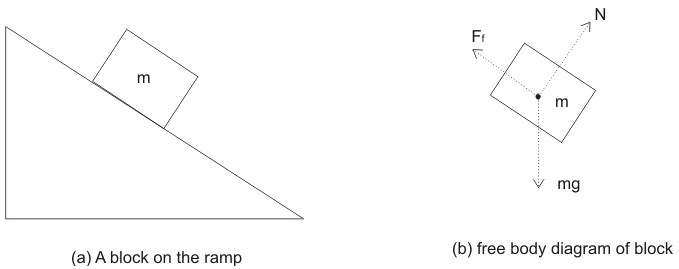1. A body in free body diagram may be subsystem of the overall system of interest.
2. The forces and moments are shown in a free body diagram at the point where they are applied.
3. Try to draw the contact forces outside the sketch of the system for viewing clarity.
4. Free body diagrams shows all external forces acting on the body and they do not show any internal forces.
5. Free body diagrams shows nothings about the motion of the system.

How to draw a free body diagram

• First create a mental picture of the body for which you want to write momentum balance equation.
• Draw rough sketch of your system showing it to be isolated from its environment.
• Place a dot in the center of the object and at this point all the forces are assumed to be acting upon.
• For every force acting on that body , draw a vector which shows size and direction of the force. each vector should start at the dot as show in the figure given above.
• Label each vector based on the type of force and remember not to include numbers and calculations.
• There are some common forces acting on an object that you should always keep in mind and they are
(a) $F_{g}=mg$, which is the force due to gravity
(b) $F_{a}$ , which is the force applied on the body
(c) $F_{f}$ , which is the force due to friction
(d) T is the force of tension

(e) N is the normal force

(f) $F_{net}$ is the net force in the system

• If the body is at equilibrium, Net  forces acting on the body should be zero . If it is moving, the net force is equal to $ma$ where m is the mass of the body and a is the acceleration of the body

Examples on Free Body Diagrams

1. Book on a table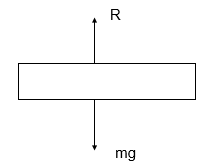mg -> Weight of the book

R -> Contact force between table and book

2. Free body diagram for a object slowing down with friction on a horizontal floor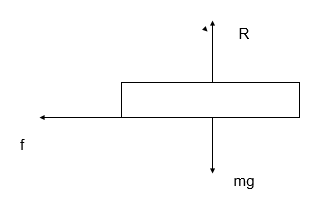mg -> Weight of the book

R -> Contact force between table and book

f -> Friction force between the object and horizontal floor

1. A set of blocks tied together by a string is being pulled by a force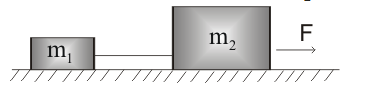Let T be the tension in the string,then free body diagrams of the blocks will be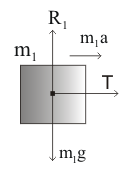R1 -> Contact force or Normal Force between block m1 and horizontal floor

m1g -> Weight of the block

T  -> Tension of the string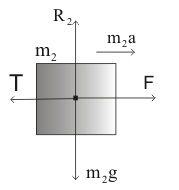R2 -> Contact force or Normal Force between block m2 and horizontal floor

m2g -> Weight of the block

T  -> Tension of the string

F  -> Force applied on the body

1. Free body diagram of Atwood Machine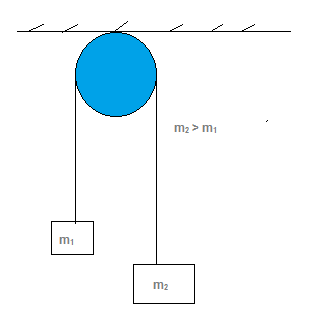Let T be the tension in the string,then free body diagram of the both the blocks will be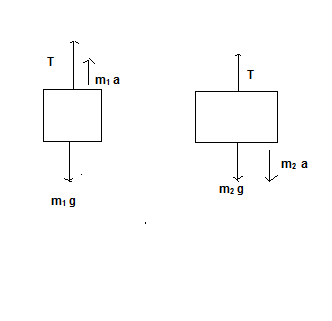Here we have learned about free body diagrams and how to draw one. So you can use this knowledge for solving problems in physics.

Quick video about Free body diagram

Practice Problems

1. Draw the free body for the object hanging from ceiling as shown below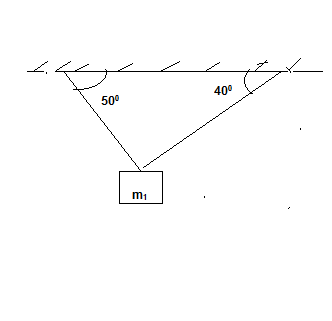2.  A person in a elevator moving up with acceleration a

3  A stone thrown upward with some velocity.

Related Articles

Fictitous Force and Free falling Elevator

Newton’s Third Law of motion (action-reaction and examples)

Solving physics problems

How to solve Newton’s Law problems

How to solve problems with Body in Static equilibrium

Different type of Forces and their origin